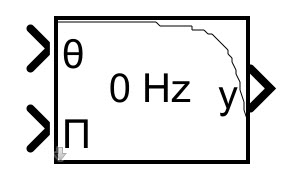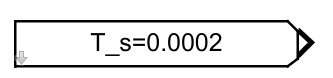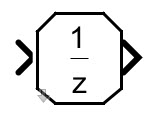Recently I discovered an undocumented feature of Simulink, i.e., plot bold line in block mask’s icon drawing commands.

### Limitations of the plot in icon drawing commands

The plot function in the mask is a reduced plotting function. If we specify the linewidth parameter to it, an error will be prompted:

Invalid arguments passed to 'plot' drawing command in block 'block_path'. Plot command accepts only numbers as valid arguments.

In the help document, the description of plot is

plot(Y) plots, for a vector Y, each element against its index. If Y is a matrix, it plots each column of the matrix as though it were a vector.

plot(X1,Y1,X2,Y2,...) plots the vectors Y1 against X1, Y2 against X2, and so on. Vector pairs must be the same length and the list must consist of an even number of vectors.

Plot commands can include NaN and inf values. When Simulink® software encounters NaNs or infs, it stops drawing, and then begins redrawing at the next numbers that are not NaN or inf. The appearance of the plot on the icon depends on the units defined by the Icon units option in the Mask Editor.

### An undocumented workaround to draw bold lines

Inadvertently, I found that if the first pair of elements of the input parameter pair of the plot is the same as the second pair of that, the plot will draw a thick line, otherwise it will draw a thinner line, i.e.,

if X1(1)==X1(2) && Y1(1)==Y1(2)
% draw bold line
else
% draw thin line
end

Therefore, we can use the plot function to draw a bold border instead of a thin border.

### Examples

#### A customized low pass filter block

x = linspace(0,1,100);
y = x./sqrt(x.^2 + 0.01);
plot(1-x,y);
plot([0 0 1 1 0 0]*0.98,[0 0 0 1 1 0]+0.02);#### A customized constant input block

pos    = get_param(gcb,'Position');
width  = max(1,abs(pos(3)-pos(1)));
height = max(1,abs(pos(4)-pos(2)));

scale  = height / width;
right  = 1 - 1/width;
bottom = 1/height;

plot([0 0 right-0.2*scale right right right-0.2*scale 0 0],[bottom bottom bottom 0.2 0.8 1 1 bottom]);#### A customized unit delay block

width  = max(1,abs(pos(3)-pos(1)));
height = max(1,abs(pos(4)-pos(2)));
scale  = height / width;
right  = 1 - 1/width;
bottom = 1/height;

dpoly(,[1 0],'z');

plot([0 0 0.2*scale right-0.2*scale right right right-0.2*scale 0.2*scale 0 0],[0.2 0.2 bottom bottom 0.2 0.8 1  1 0.8 0.2]);